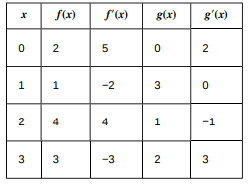# ?For the following exercises, use the information in the following table to find h?(a)

Chapter 3, Problem 246

#### (choose chapter or problem)

For the following exercises, use the information in the following table to find h′(a) at the given value for a.h(x) = g( f(x)); a = 0

Unfortunately, we don't have that question answered yet. But you can get it answered in just 5 hours by Logging in or Becoming a subscriber.